## 播客

]]>
<![CDATA[MRI序列]]> tag:https://laffitto.xyz,2022-07-17:mrisequence 2022-07-17T17:29:52+08:00 2022-07-17T17:29:52+08:00 laffitto https://laffitto.xyz 工作中要用到MRI的一些知识，这里先做一些简单的整理，后续会持续整理更新。

## 术语描述

### 强度/intensity

• high signal intensity = white
• intermediate signal intensity = grey
• low signal intensity = black

• hyperintense = brighter than the thing we are comparing it to
• isointense = same brightness as the thing we are comparing it to
• hypointense = darker than the thing we are comparing it to

tips： CT中描述强度使用density，避免混淆使用！

## MRI序列

MRI仅能探测横向宏观磁化矢量。而氢质子进入高能状态后，可以产生此类矢量。

### T1加权/T1 weighted

• 脂肪：白色 high signal intensity

• 肌肉：灰色 intermediate signal intensity

• 流体（尿液，脑脊液）：深色 low signal intensity

• 流动的血液：深色 low signal intensity

• 骨：深色

• 空气：深色

• 脑：

• 灰质：灰色

• 白质：明亮（与灰质相比高信号）hyperintense compared to grey matter (white-ish)

T1 最适合用于评估解剖结构，因为图像在宏观上类似于组织。

### T2加权/T2 weighted

• 脂肪：白色 high signal intensity
• 肌肉：灰色 intermediate signal intensity
• 流体（尿液，脑脊液）：白色 high signal intensity
• 流动的血液：深色
• 骨：深色
• 空气：深色
• 脑：
• 灰质：灰色
• 白质：灰暗（与灰质相比低信号）hypointense compared to grey matter (dark-ish)

#### Short Tau Inversion Recovery (STIR)

STIR 不能在注射钆后使用，因为钆的 T1 在相同的脂肪范围内，最终来自它的信号会减弱。

• 脂肪：深色
• 肌肉：比脂肪更黑
• 流体：非常明亮
• 骨：深色
• 空气：深色
• 脑：
• 灰质：灰色
• 白质：比灰质更暗

• 脂肪：白色
• 肌肉：灰色
• 流体：白色
• 骨：深色
• 空气：深色
• 透明软骨：灰色
• 纤维软骨：深色

### 扩散加权序列

• 液体（如尿液、脑脊液）：不限制扩散
• 软组织（肌肉、实体器官、脑）：中间扩散
• 脂肪：由于缺水，信号微弱

#### 传统DWI/diffusion weighted image（DWI）

DWI 专门检测水分子中质子的运动。它代表了实际扩散值和 T2 信号的组合。

• 脂肪：由于缺水，信号微弱
• 肌肉：灰色
• 流体（CFS）：深色
• 脑：
• 灰质：灰色
• 白质：灰暗（与灰质相比略低）

• CSF：高信号（白色）
• 脂肪：由于缺水，信号微弱
• 其他软组织：中等信号强度（灰色）
• 灰质：中间信号强度（灰色）
• 白质：与灰质相比略高

## 参考文章

]]>
<![CDATA[青岛之旅]]> tag:https://laffitto.xyz,2022-06-26:qindaojourney 2022-06-26T11:44:57+08:00 2022-07-03T20:36:49+08:00 laffitto https://laffitto.xyz 工作了之后就没有时间了，本着及时行乐的态度，毕业后赶紧跑回家，来一趟轰轰烈烈的毕业旅行。

## 基本准备## 沿途景色

### 青岛海昌极地海洋公园### 青岛啤酒博物馆### 崂山风景区### 小麦岛### 城市中的美景### 帆船### 五四广场灯光秀## 吃吃喝喝## 城市印象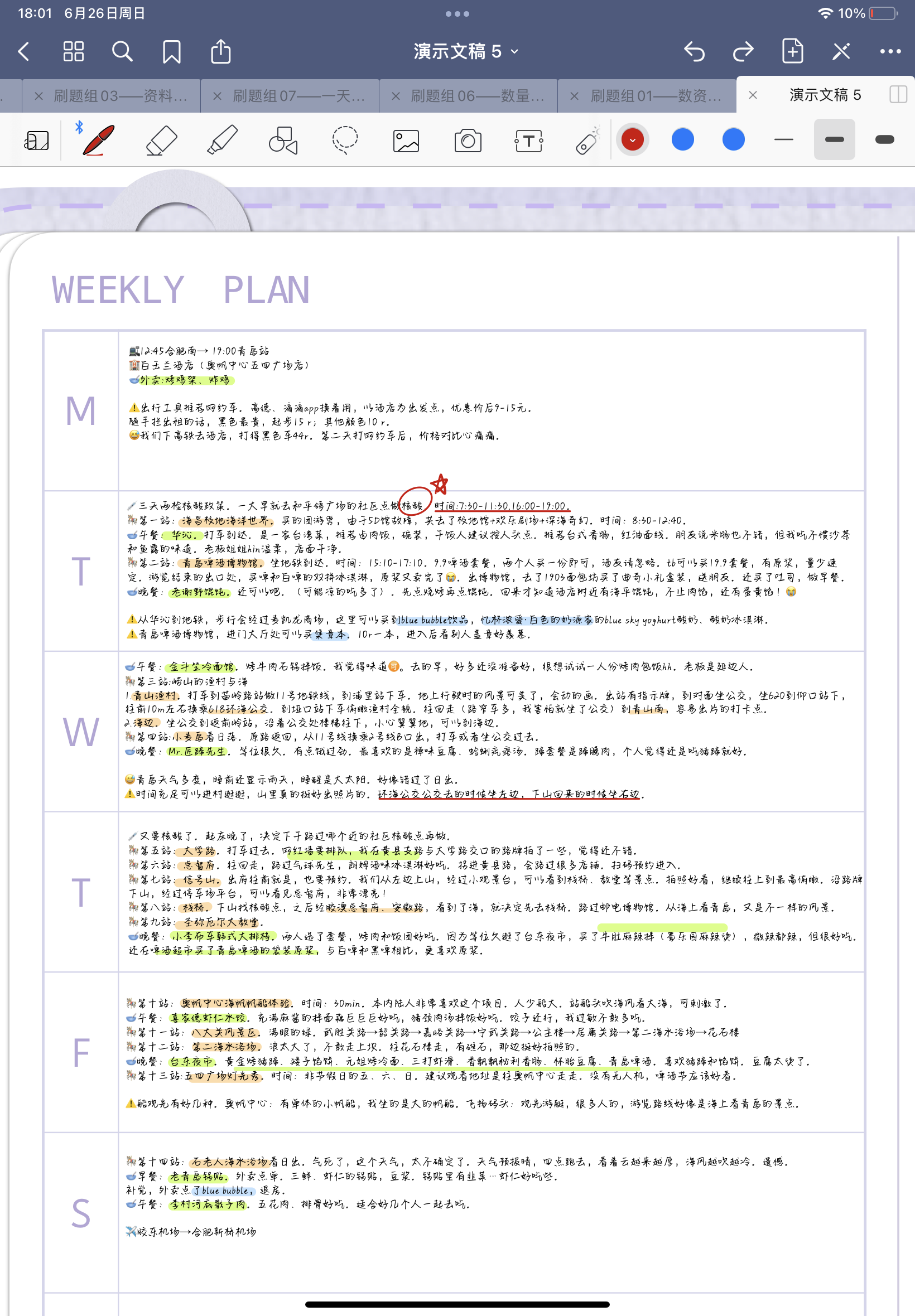]]>
<![CDATA[毕业]]> tag:https://laffitto.xyz,2022-06-13:bi-ye 2022-06-13T20:05:04+08:00 2022-06-21T20:05:32+08:00 laffitto https://laffitto.xyz 当整理着自己一份又一份的毕业材料时，我终于明白，自己的求学生涯要迎来终点了。]]>
<![CDATA[「写作建议」笔记]]> tag:https://laffitto.xyz,2022-03-31:写作建议笔记 2022-03-31T11:07:40+08:00 2022-03-31T11:19:28+08:00 laffitto https://laffitto.xyz 博客也写了很久了，但是很多时候还是感觉在盲人摸象。很多时候脑袋一热，一口气写完了文章，潦潦草草就发布了，反过来自己都不愿意看。虽然说是记录自己生活与感想的博客，但也不能太过于随性。有时候也想改进自己的写作水平，但始终无从下手。

## 明确文章的起点与终点

### 写作的终点：明确文章的目标

• 这篇文章传达什么信息？
• 这篇文章中的「我」是谁？
• 哪些人会看这篇文章？这篇文章中写给谁？

• 读者的知识背景

• 读者对文章的期待和态度

• 列数字、做对比
• 通过具体的故事来制造冲突

## 修改文章

### 检查文章主题

• 文章涉及范围越写越大；
• 每个环节堆积了或重复、或与主题无关的素材。

### 改善语言风格

• 避免语言上的「炫技」。文章要以通俗易懂、没有语病为最高目标。
• 避免口语化的表达。过多口语表达会显得文章专业性不够。
• 避免所谓的「大词」。指一些无法传递出实质意义的词汇，容易自嗨。

## 个人感想

1. 选题。平时收集记录有意思的东西作为材料库。选好内容后确定我这篇文章的主题，用一句话总结想写的内容。并明确文章定位。
2. 列大纲。根据不同主题罗列出对应的大纲。以把握好全局内容架构。
3. 书写补充正文。根据大纲完成文章。
4. 修改文章。从文章主题，行文节奏，语言风格上修改文章。
5. 发布。发表到blog等其他平台上。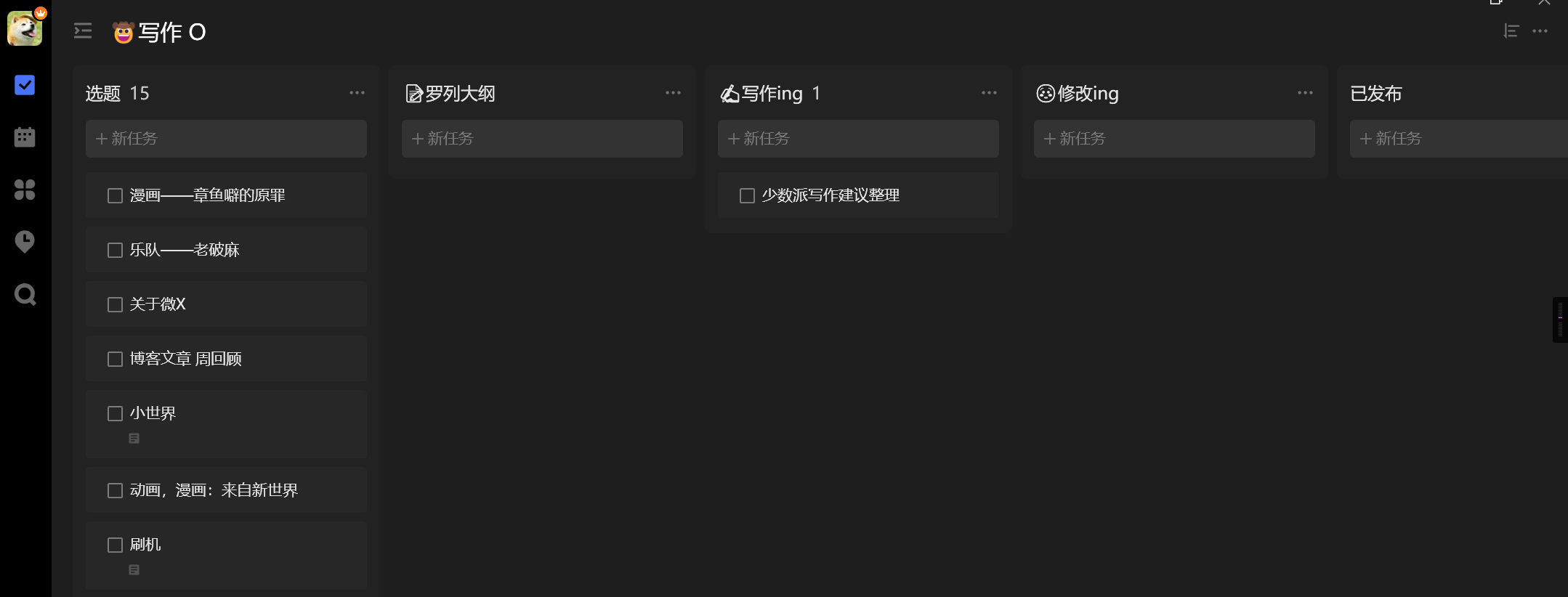]]>
<![CDATA[生日快乐]]> tag:https://laffitto.xyz,2022-03-05:sheng-ri-kuai-le 2022-03-05T12:17:48+08:00 2022-03-05T12:17:48+08:00 laffitto https://laffitto.xyz 最近一段时间都要忙毕业论文，之前想写的东西一直也拖着。不过2月25号过完了自己25岁的生日，感觉还是挺奇妙与值得留恋的。再此记录留念一下。]]>
<![CDATA[鸟生]]> tag:https://laffitto.xyz,2022-01-17:niao-sheng 2022-01-17T23:18:36+08:00 2022-01-17T23:18:36+08:00 laffitto https://laffitto.xyz 咱家喜欢养点宠物，但受限于房间的大小，大多数都是些小动物，而这其中要属虎皮鹦鹉是最多的。为什么说是最多呢，因为它们经历了一代又一代。最开始是一对，然后死掉了一只，再买只新的替补，就这样往往复复，除了中间有两只鸟儿相继死去，断了大概两年。从小学到研究生，基本贯穿了我整个读书的生涯。

『老妈的微信头像之超级远古稚嫩：他还是个孩子呀！』『偷拍洗澡』『挠痒痒呢』『一路走好，谢谢陪伴』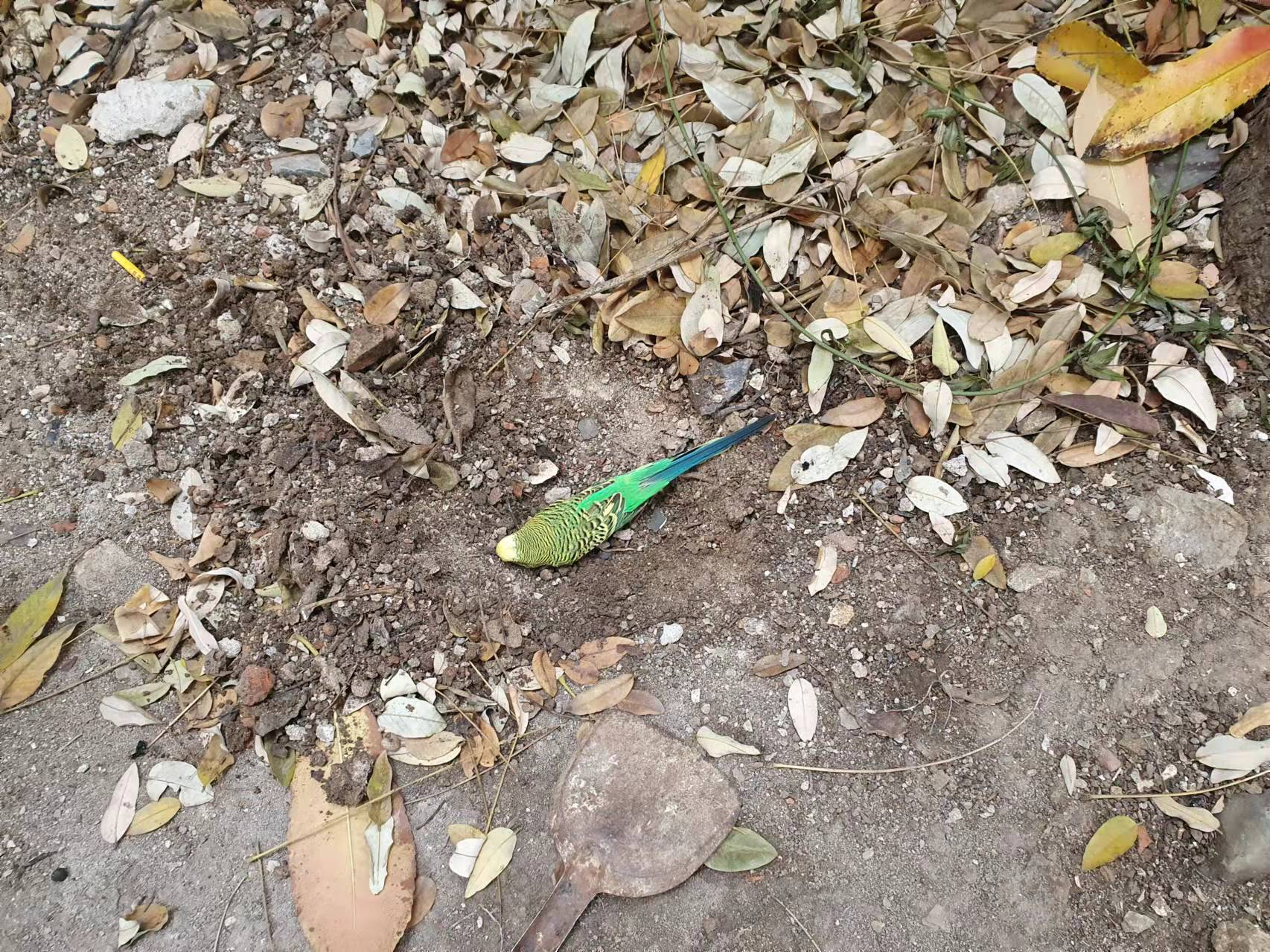]]>
<![CDATA[2022 Flag]]> tag:https://laffitto.xyz,2022-01-03:2022flag 2022-01-03T20:05:38+08:00 2022-01-03T20:05:38+08:00 laffitto https://laffitto.xyz 新的一年，新的Flag！

1. 阅读计划：失败，目标6本，仅完成2本，还是没有形成阅读流。
2. 写作计划PLUS：计划2周一篇，实际完成23篇，勉强完成，仍需努力。
3. Girlfriend：成功找到我的可爱小朋友，不错不错。
4. 论文发表：失败，离谱。
5. 算法和编程：这个终点事件是找到工作。工作是找到了，但仍然需要努力精进自己的技术，算完成一半吧。
6. 保持健康：额，在学校的日子都挺自律的，实习上班的日子有点怠惰，流程还需要优化。
7. 投资长跑：没啥钱，但还是跟投了，后面也没啥时间管，完成的较失败，到时候做一下复盘。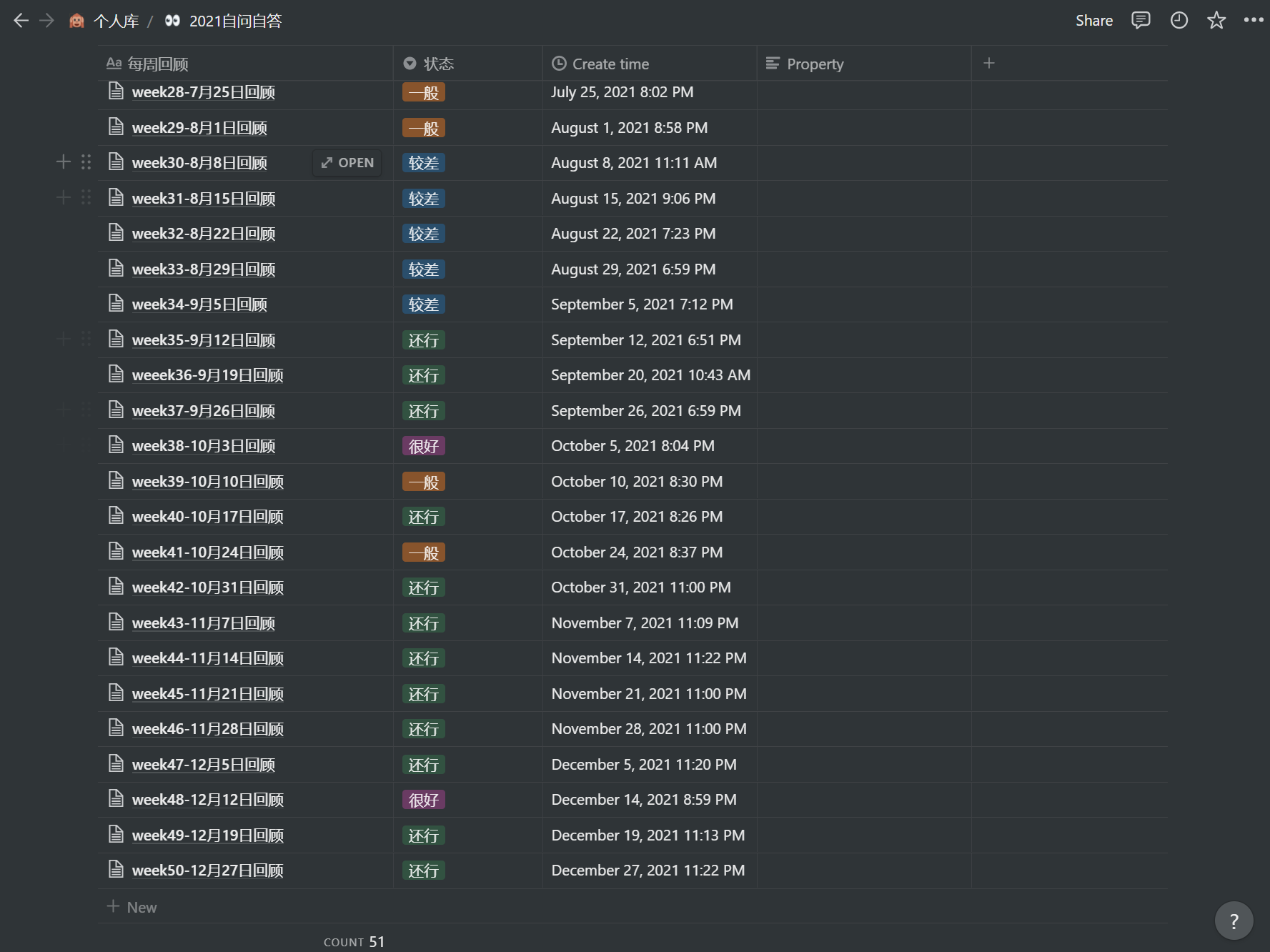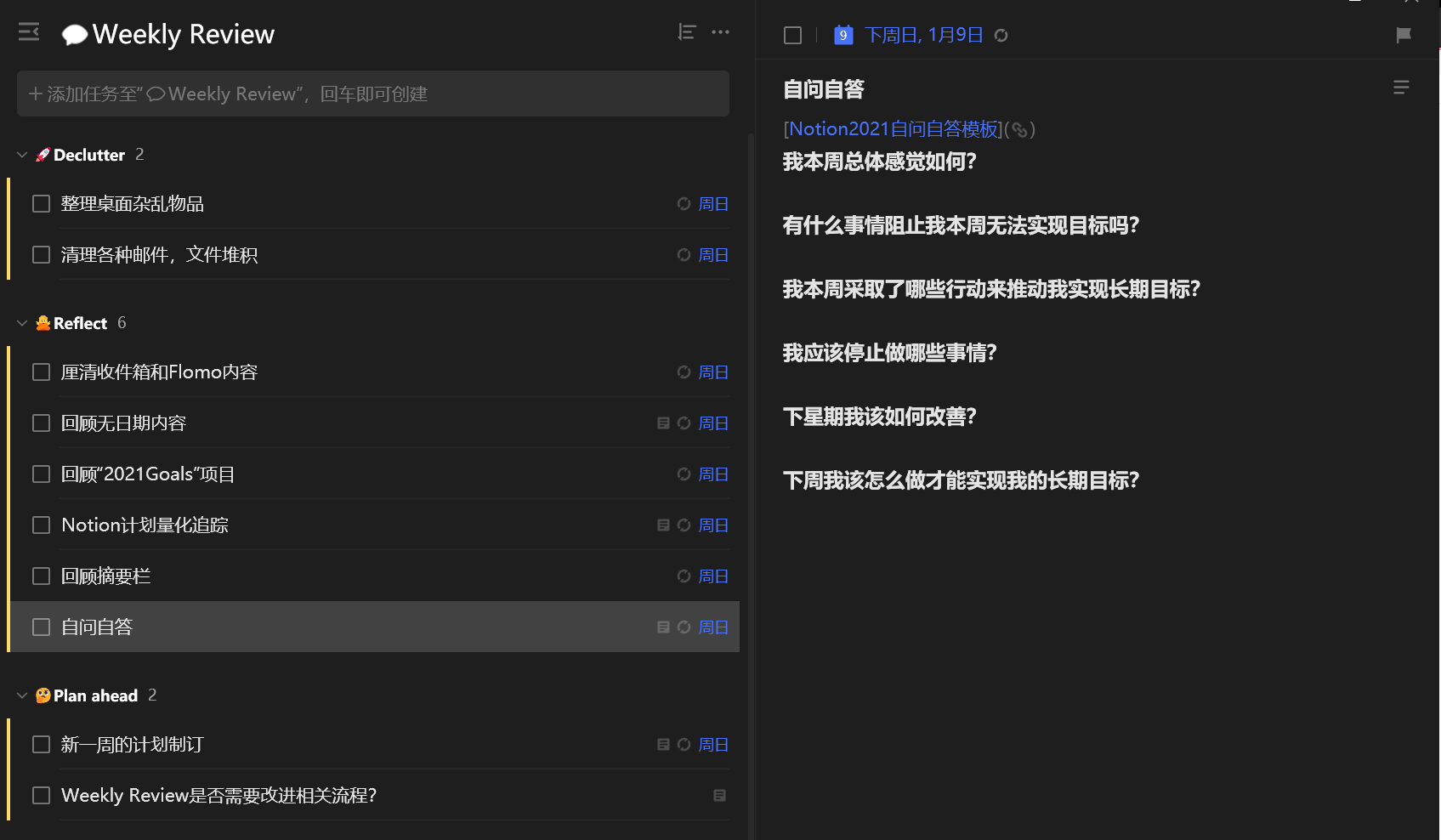# 2022 Flag

## 愿望方面

### 长期投资

]]>
<![CDATA[2021年终总结]]> tag:https://laffitto.xyz,2022-01-03:2021-nian-zhong-zong-jie 2022-01-03T17:59:18+08:00 2022-01-03T18:01:08+08:00 laffitto https://laffitto.xyz 总体来说，2021年过得差强人意。这一年三个重要的关键词是爱情，工作，论文。

### 关于爱情### 关于工作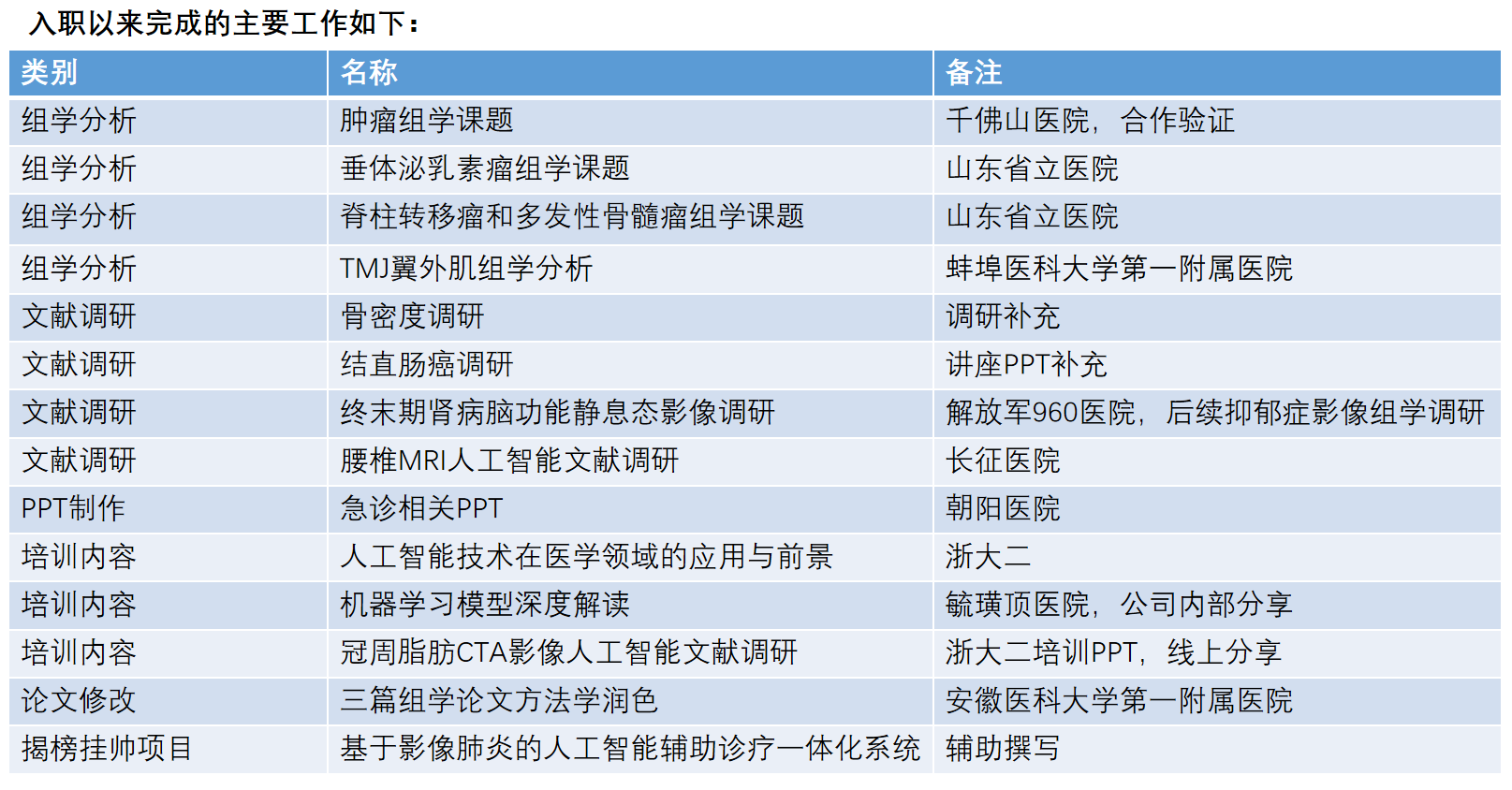### 关于生活]]>
<![CDATA[济南]]> tag:https://laffitto.xyz,2021-11-21:ji-nan 2021-11-21T19:21:07+08:00 2021-11-21T19:21:07+08:00 laffitto https://laffitto.xyz 由于实习工作原因暂时来到了山东济南。本身对于山东有一种莫名的亲切感，可能是姥姥的老家缘故，也可能是之前接触的山东人都充满了好感。突然就有一种落叶归根的感觉。

## 玩## One more]]>
<![CDATA[实习新挑战！！]]> tag:https://laffitto.xyz,2021-10-31:shi-xi-xin-tiao-zhan- 2021-10-31T21:10:08+08:00 2021-10-31T21:10:08+08:00 laffitto https://laffitto.xyz 这一周，正式开始工作前的实习了。

]]>
<![CDATA[软件-Flomo]]> tag:https://laffitto.xyz,2021-10-17:ruan-jian-flomo 2021-10-17T19:48:36+08:00 2021-10-17T19:48:36+08:00 laffitto https://laffitto.xyz 最近开始使用一个Flomo的软件。软件的内容实际很简单，它是一个随机记录灵感的软件。最开始的时候它并没有引起我的注意，因为我觉得如果只是单纯记录灵感的话，我有着更多的替代品。完全没有必要去花费精力与金钱去使用一个新的软件。然而在了解了这个软件背后的哲学后，又激发起了我对它的兴趣。

Flomo官网的介绍说自己是新一代的「卡片笔记」。其目标关注于极简输入，方便管理，定期回顾三个方面。其背后的哲学依据是根据卢曼的卡片笔记法。卢曼这个人用他创造的卡片式笔记写作法完成出版了58本著作和数百篇文章。这是一套方法，有着完备的方法论，而Flomo就是基于这套方法论，结合对现今世代的思考研发出的这么一款产品。看过作者的访谈，开发团队不过两个人，但是它们的想法很好，有着自己产品的立场和设计理念。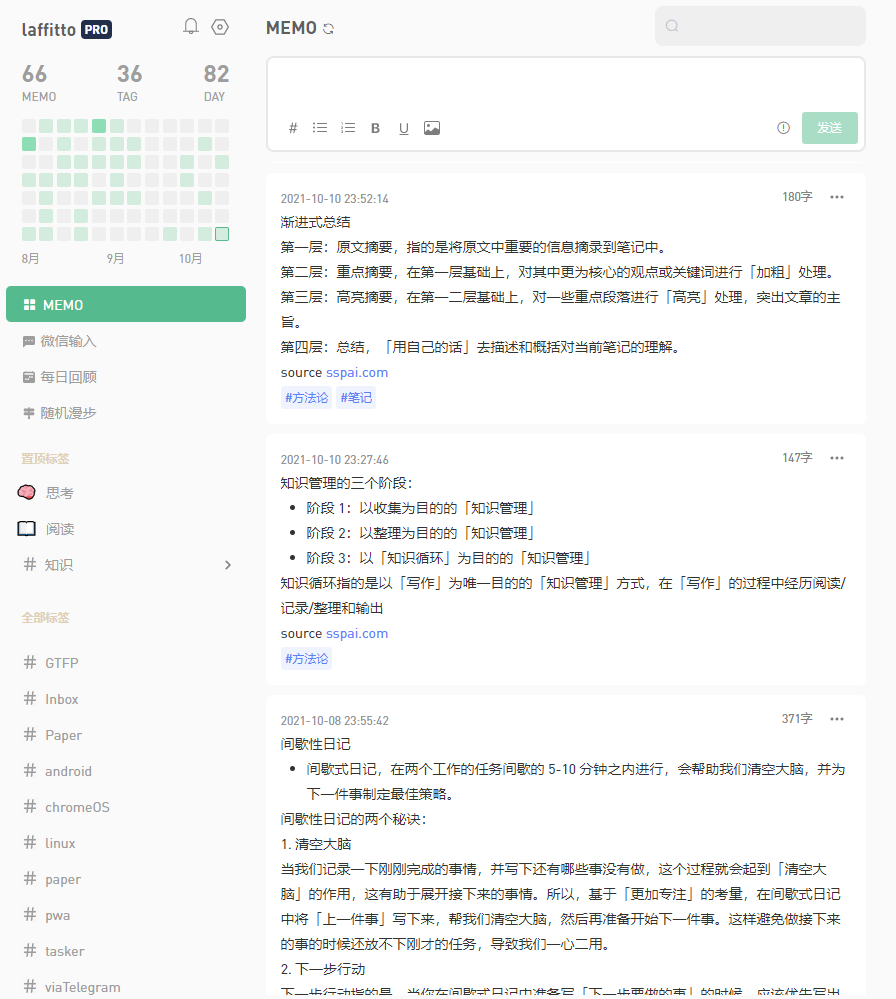]]>
<![CDATA[长大的我们]]> tag:https://laffitto.xyz,2021-10-05:chang-da-de-wo-men 2021-10-05T16:42:22+08:00 2021-10-07T16:43:12+08:00 laffitto https://laffitto.xyz 很久没有更新博客了，沉寂了大概一个多月。不是说没有时间，只能说在周围大环境的推动和焦虑的心情下，我选择了去处理重要且紧急的事情。其他时间只想放空自己，让自己正常运转。好在已经处理完成大部分事务，又重新回来了。

]]>
<![CDATA[双拼 for Chrome OS]]> tag:https://laffitto.xyz,2021-08-16:shuangpinchromeos 2021-08-16T09:20:29+08:00 2021-08-16T09:53:17+08:00 laffitto https://laffitto.xyz 最近在闲鱼上入了一个pixel slate，基于Chrome OS的二合一平板电脑。Chrome OS可能很多人都没听过，它是Google基于chrome主要运行web程序的系统。早些时期基本就是个浏览器，在Google近几年不断完善更新下，现在的Chrome OS可以看作是Chrome browser+PWA应用+Android+Linux的缝合产物。

## 平板模式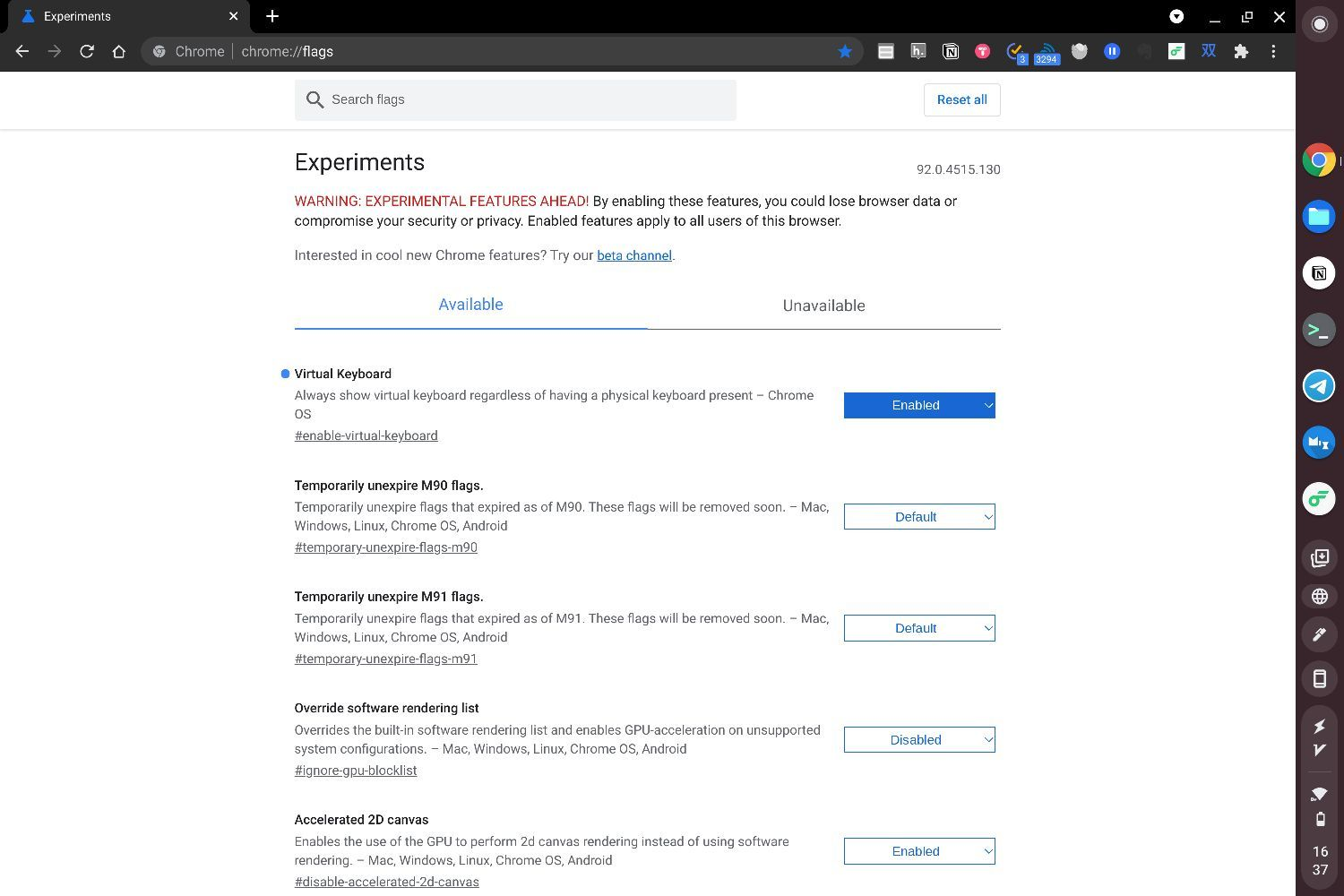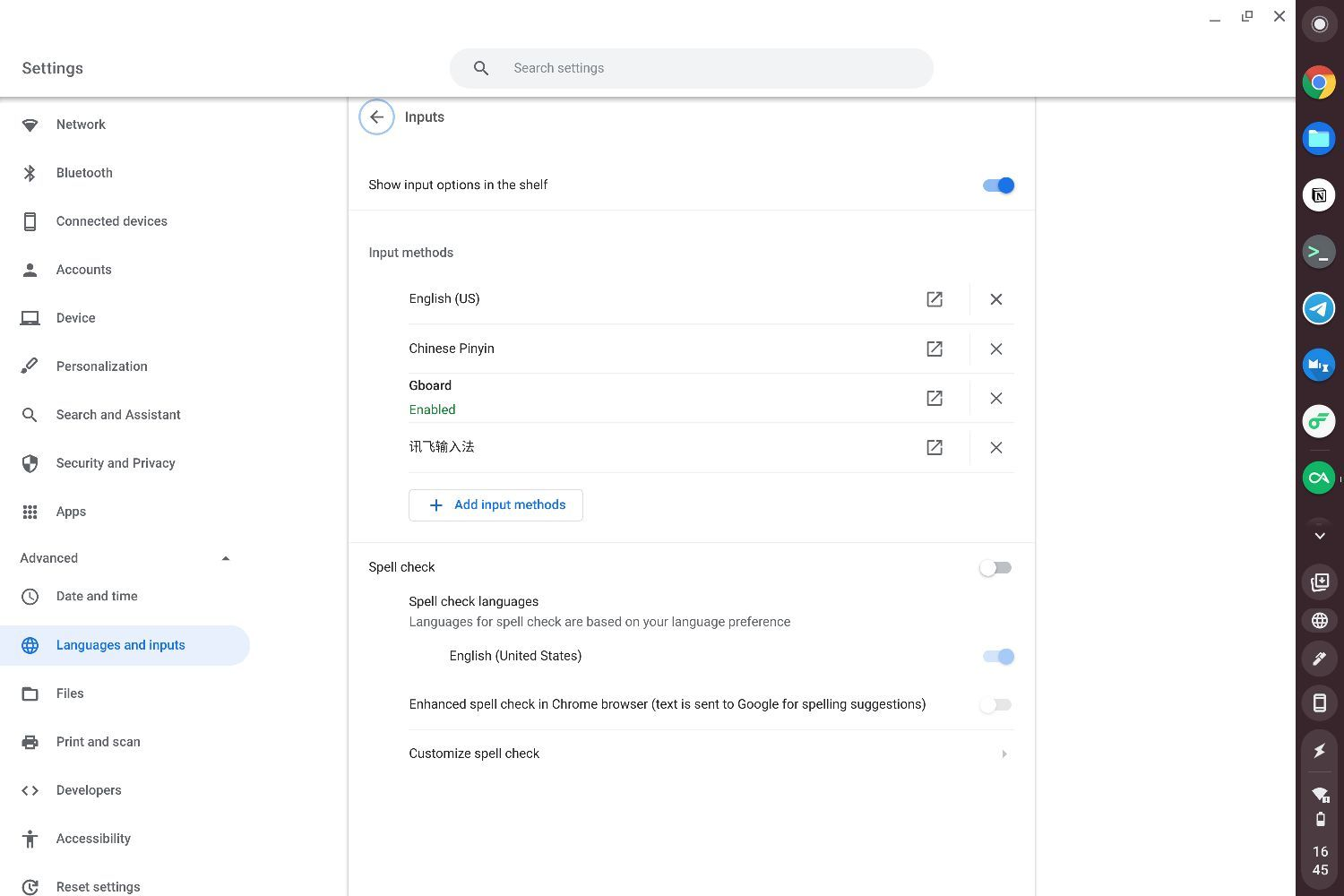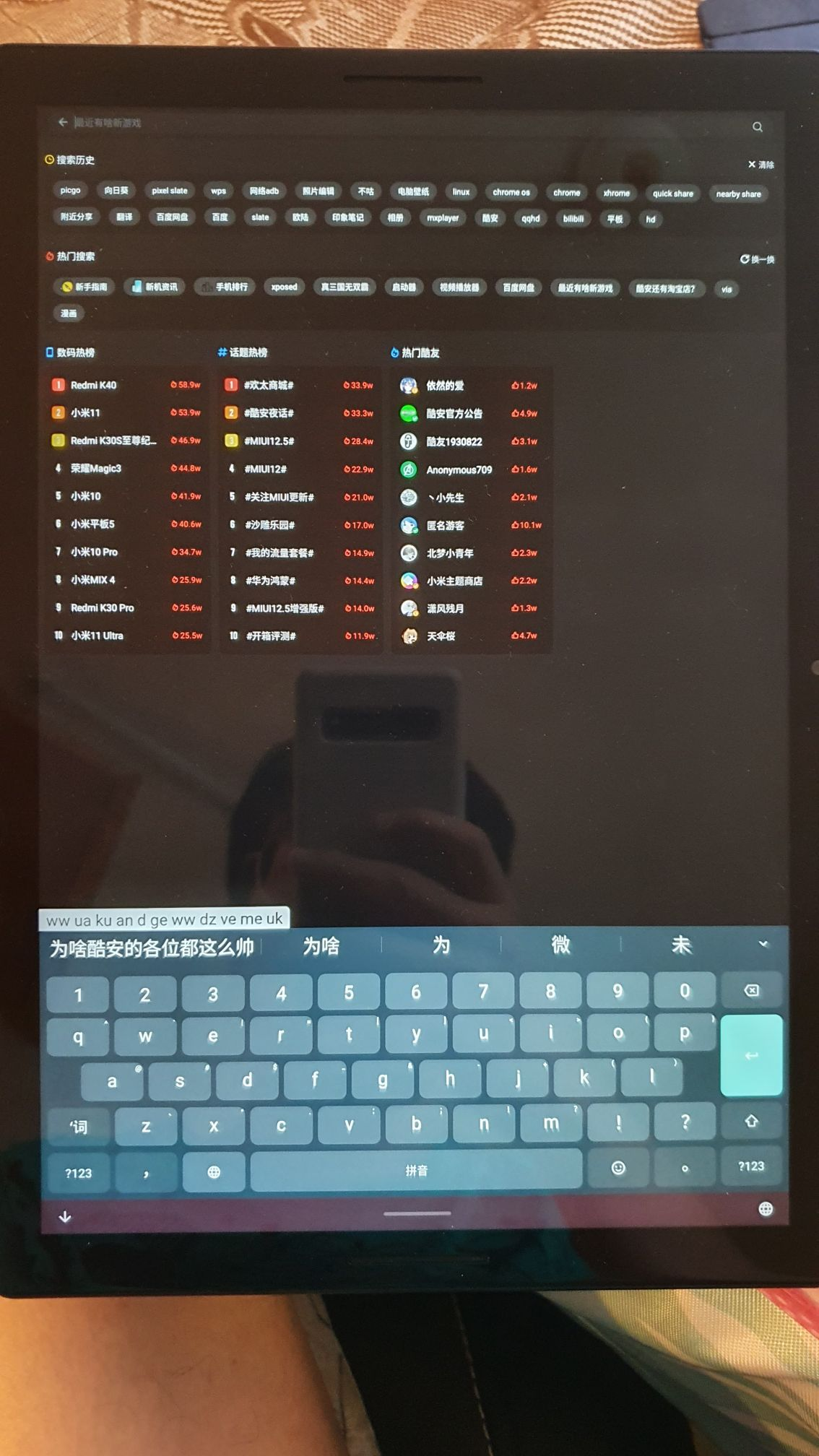## 电脑模式

### Android应用

Android借助之前安装的第三方的输入法。由于在平板模式下的设置，即便切换到电脑模式，输入的时候也会有虚拟键盘弹出，这里我使用了Gboard自带的悬浮键盘，缩小一下比例，也算勉强能用了吧（假装看不见下面的东西）。打字能够在输入法中显示，并且上屏。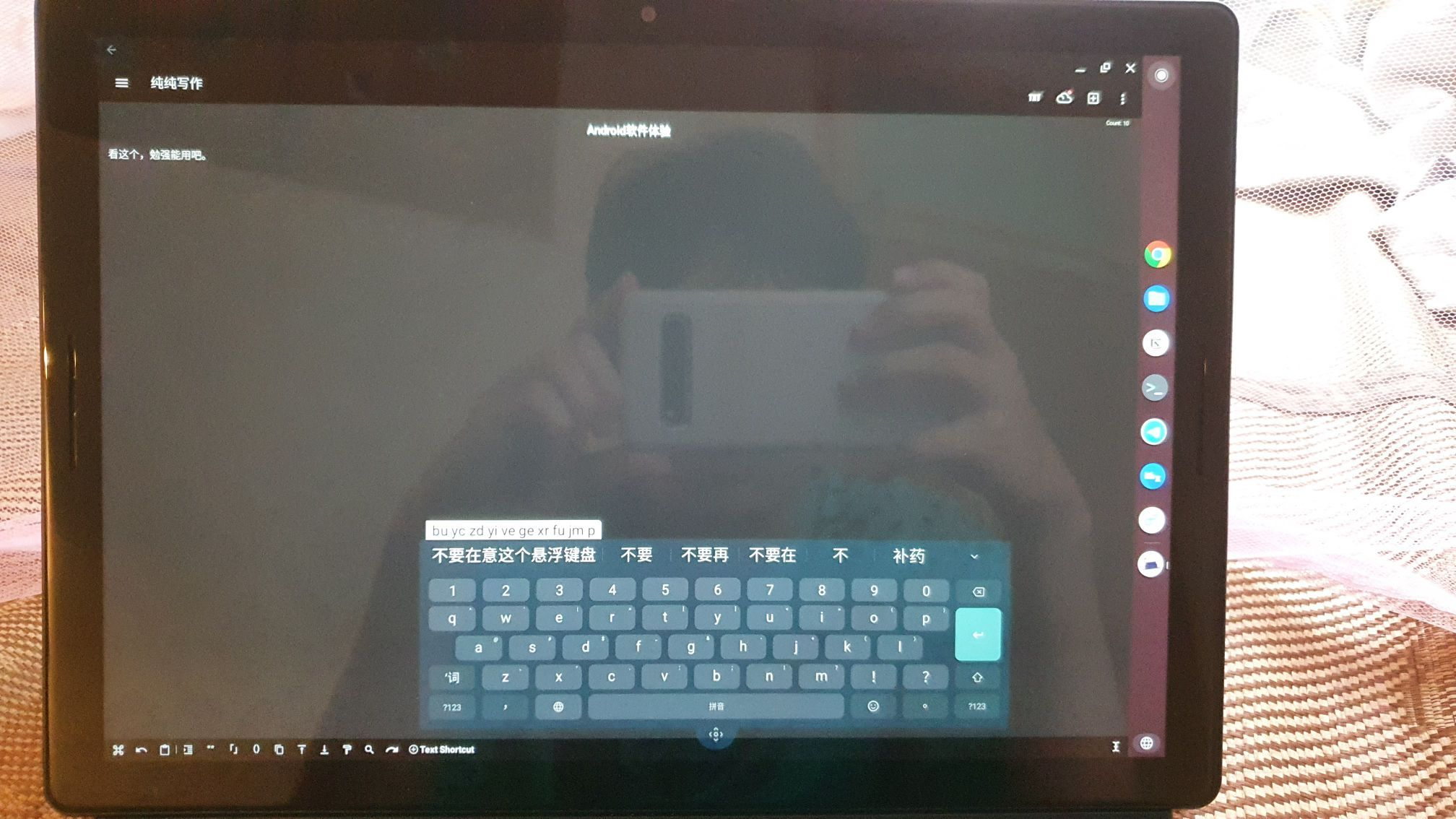### Chrome Browser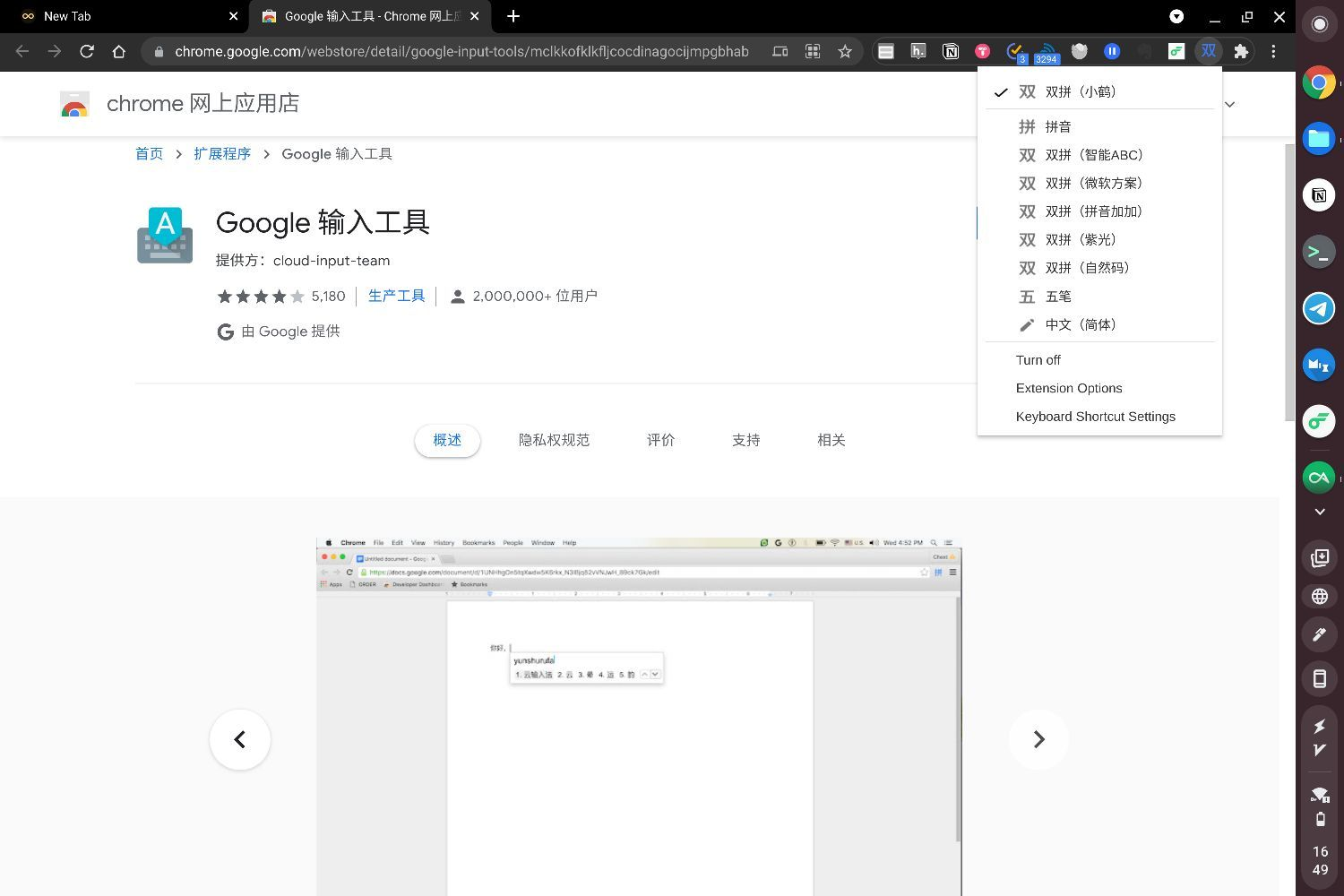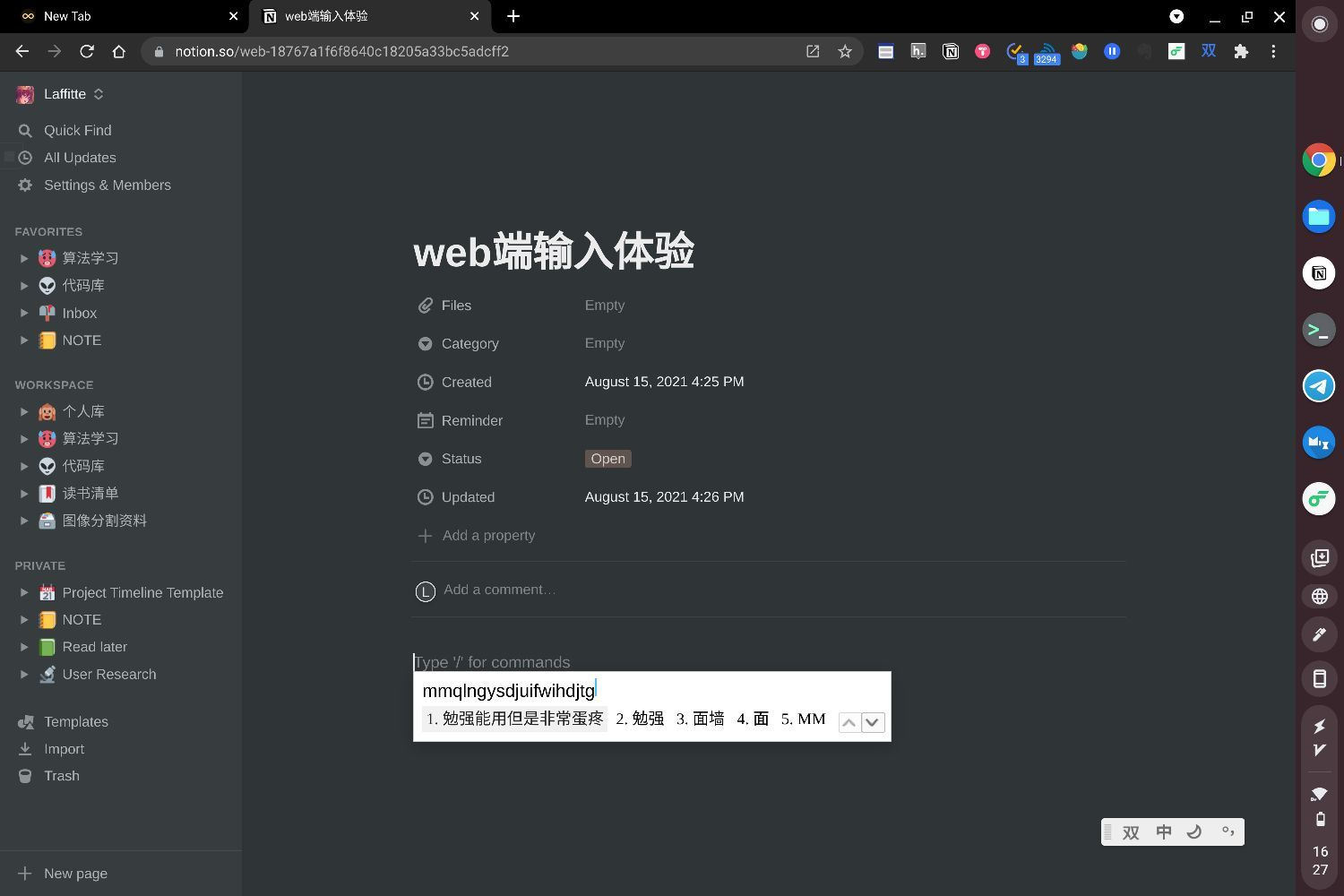### Linux应用

Chrome OS可以安装Liunx软件，还是挺有意思的，不过这个情况下无法使用android第三方输入法。不过那样可以选择使用linux的双拼输入法呀。

1. 安装中文字体

sudo apt install fonts-wqy-microhei fonts-wqy-zenhei

sudo dpkg-reconfigure locales

sudo reboot
1. 安装Fcitx输入工具

sudo apt update && sudo apt upgradesudo apt-get install fcitx -ysudo apt-get install fcitx-lib* -y
1. 配置输入设备

1. 启用中文输入法
• 终端输入fcitx开启服务（非常重要！不然可能会发现没有任何输入法选项，之前在这卡了好久）
• 输入fcitx-configtool并回车
• 在弹出窗口中找到「双拼(Shuangpin)」并选中
• 在弹出窗口的底部菜单栏中找到「向上箭头」图标，多次点击，将「双拼(Shuangpin)」移至第二位
• 关闭弹出窗口

（这一步也可以后面再做，因为后面还需要安装输入法）

1. 配置输入法环境

sudo vi /etc/systemd/user/cros-garcon.service.d/cros-garcon-override.conf

Environment="GTK_IM_MODULE=fcitx"Environment="QT_IM_MODULE=fcitx"Environment="XMODIFIERS=@im=fcitx"

1. 双拼输入法启用

sudo apt install fcitx-module-cloudpinyin

CloudPinyin的默认云服务器是Google，如果想改成baidu，输入fcitx-configtool并回车。在Addon——Cloud Pinyin——Configure，将Cloud Pinyin Source选择为Baidu。其他的输入方式设定也可以在Addon中设定，例如我这里的Pinyin方式设为的是小鹤，完美达成目的。还有很多输入方式可以参考这个Fcitx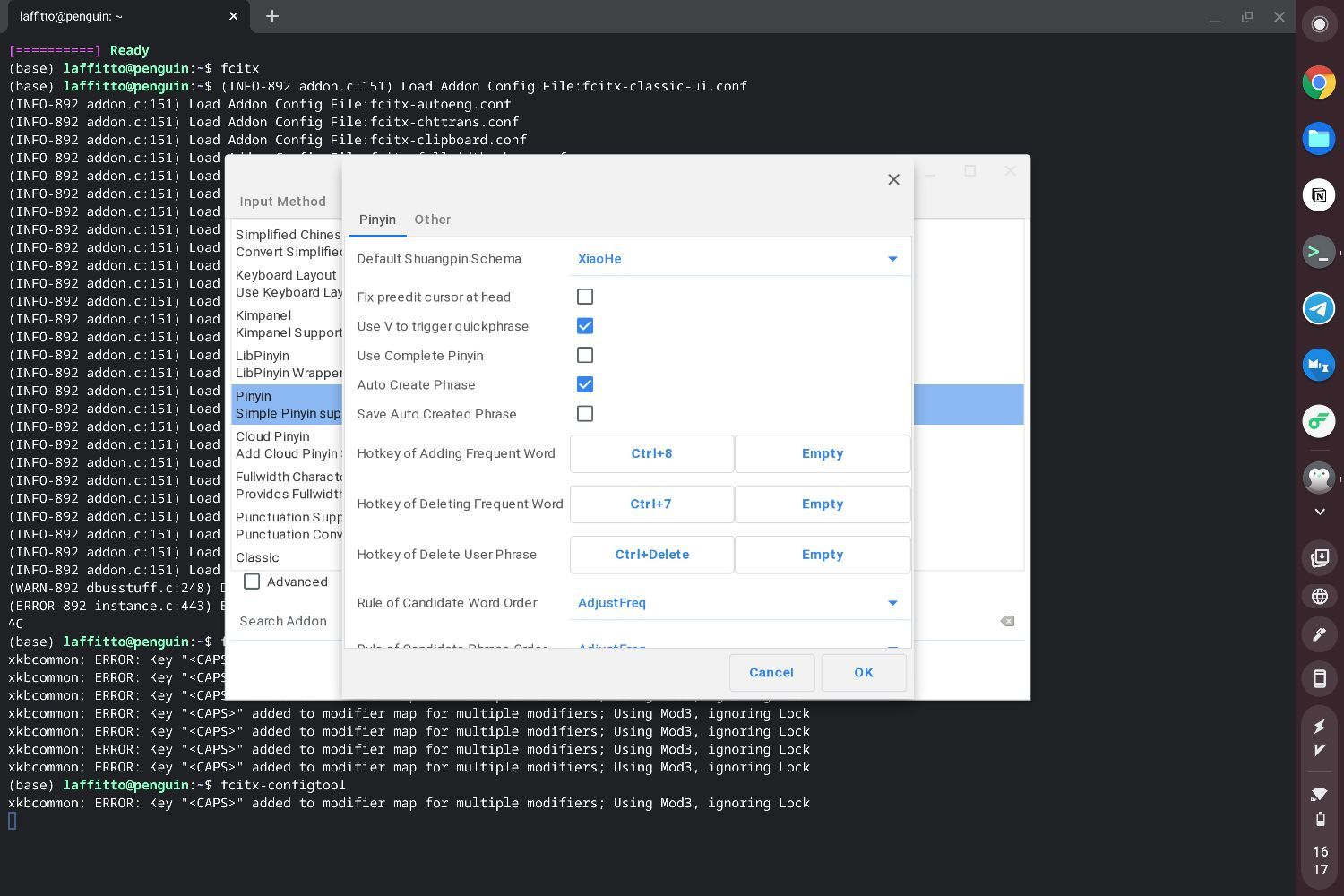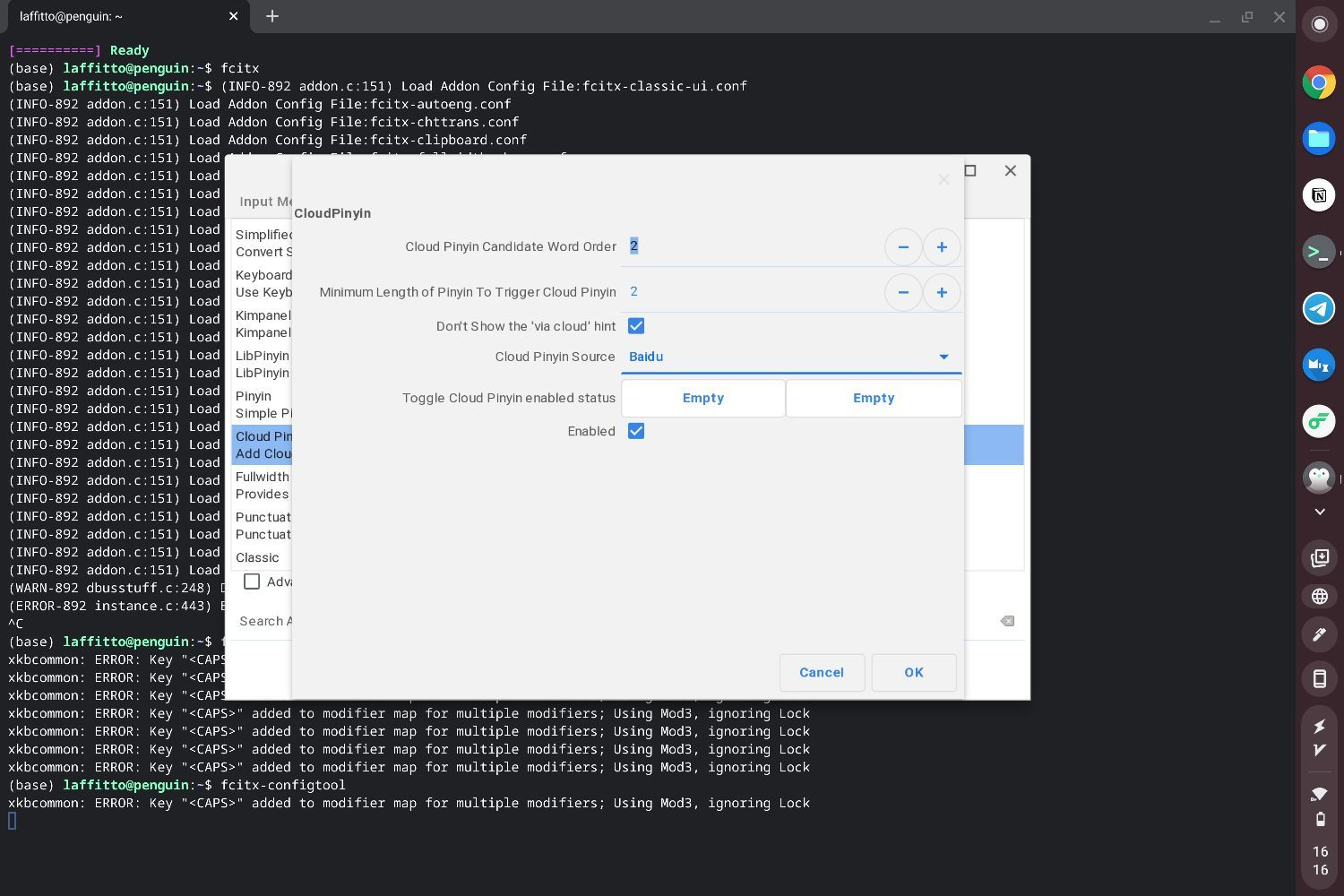sudo vi ~/.sommelierrc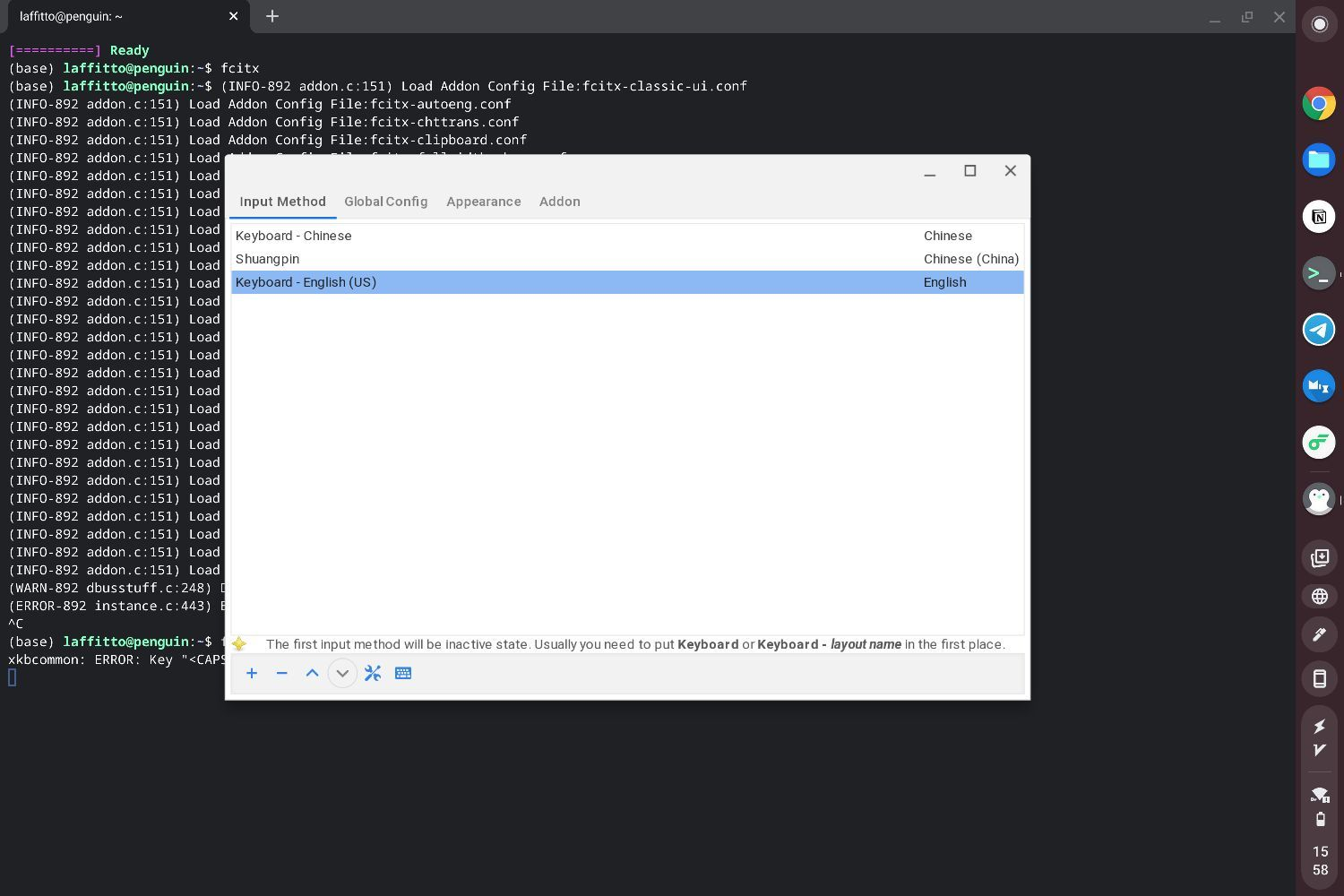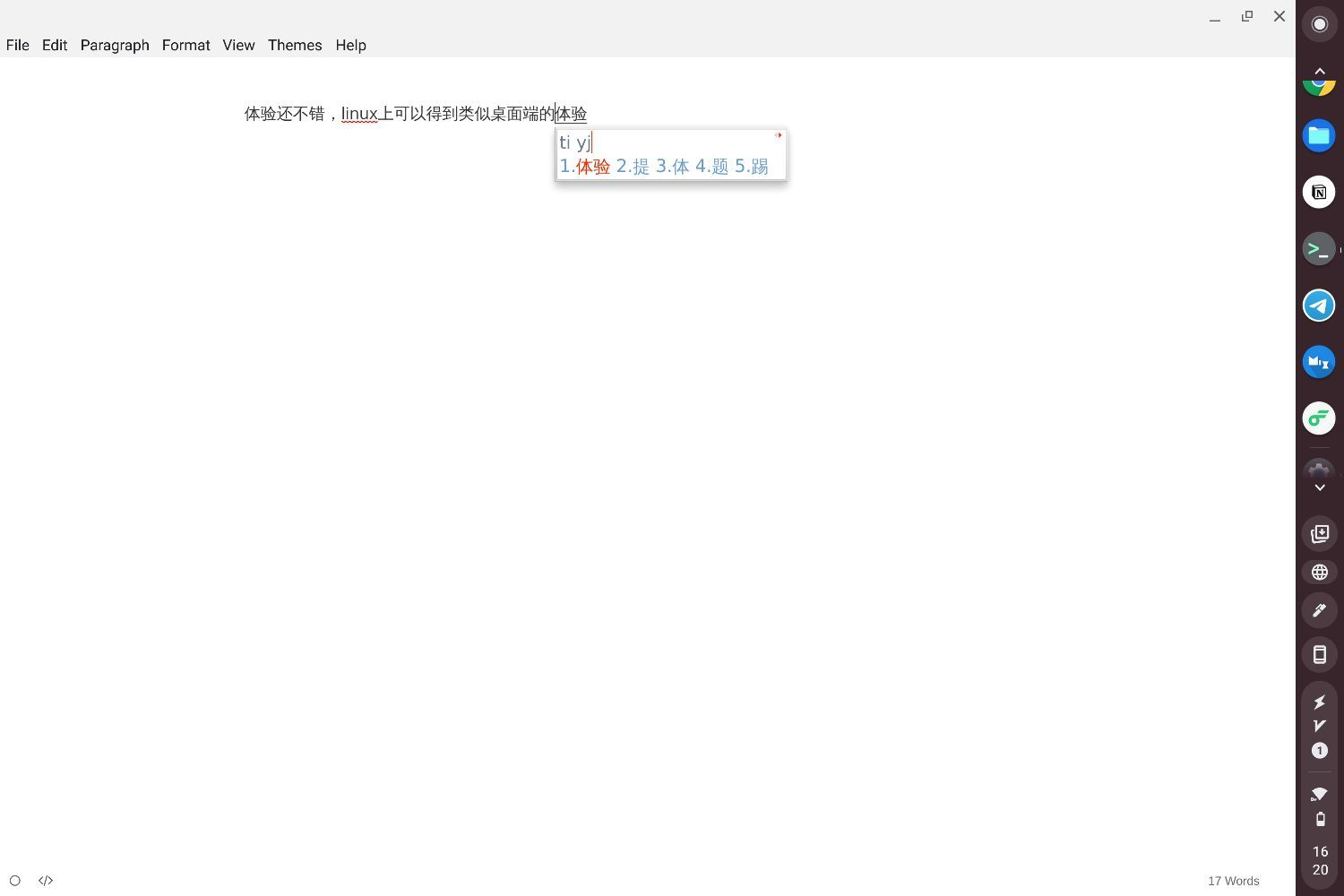]]>
<![CDATA[变狗！！！]]> tag:https://laffitto.xyz,2021-08-03:变狗 2021-08-03T22:55:20+08:00 2021-08-04T23:13:24+08:00 laffitto https://laffitto.xyz 在互联网上

]]>
<![CDATA[慎独]]> tag:https://laffitto.xyz,2021-07-18:shen-du 2021-07-18T21:49:09+08:00 2021-07-18T21:49:09+08:00 laffitto https://laffitto.xyz 专注力好像不如以前了，反思ing

​ ——礼记·中庸

「慎独」一词主要是指一个人在独处的时候，即使没有人监督，也能严格要求自己。原意中严格要求自己主要指的遵守一些道德准则，不做不道德的事情。但是现在我想表达的「慎独」，是指严格要求自己的一些很多行为，里面不仅包含了道德层面的东西，也包含了个人发展和精神层面的东西。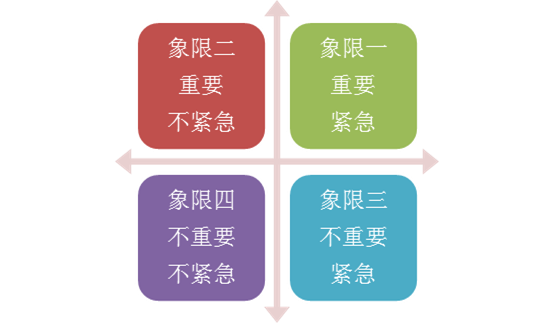]]>
<![CDATA[陷阱]]> tag:https://laffitto.xyz,2021-07-04:xian-jing 2021-07-04T21:03:14+08:00 2021-07-04T21:03:14+08:00 laffitto https://laffitto.xyz 我们经常在无知的时刻做出重要的决定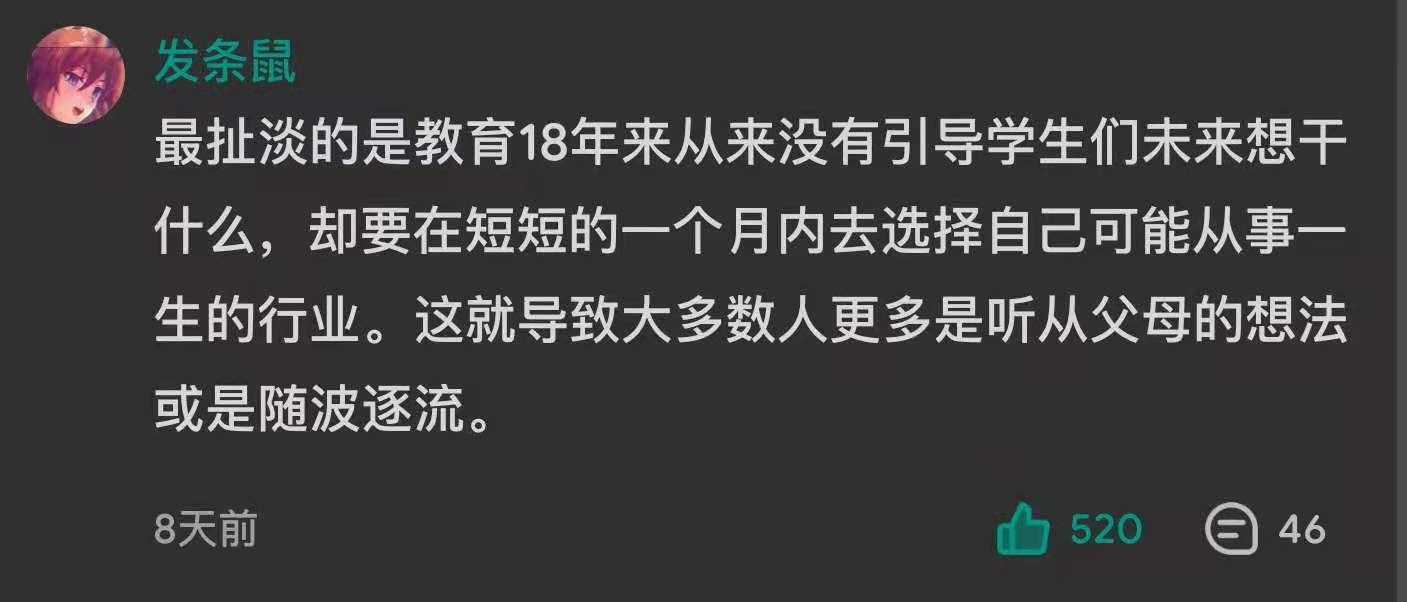18 岁在对学科与知识一无所知的时候就被要求选择自己的专业；

22-25 岁在对商业世界运行规则毫无概念的时候就被要求选择工作方向；

25-28 岁在对自己和人际关系一知半解的情况下就被要求确定长期伴侣；

]]>
<![CDATA[新博客系统探索——动态博客Halo]]> tag:https://laffitto.xyz,2021-06-20:新博客系统探索动态博客halo 2021-06-20T20:13:32+08:00 2021-06-27T20:17:16+08:00 laffitto https://laffitto.xyz 有点想迁移博客的想法

Ghost是看了少数派的推荐文章，说实话第一次看到还挺心动的，因为界面真的很好看。但是发现localhost有点问题，而且发布什么的不太方便，总体感觉有点太重了，我并不需要邮件管理订阅什么之类东西，于是暂时放下了，有时间再摆弄。

Halo是无意中翻到的，虽然想试试wordpress，但是很多评论说太重了，我也就没去尝试了，但是在体验了Halo后，感觉神清气爽，就决定是它了，新的简陋的博客展示

## 安装过程

### Docker安装失败

CentOS 8 中安装 Docker 会出现一些不兼容的问题，可以参考CentOS 8 中安装 Docker 出现和 Podman 冲突的问题解决。

## Halo特性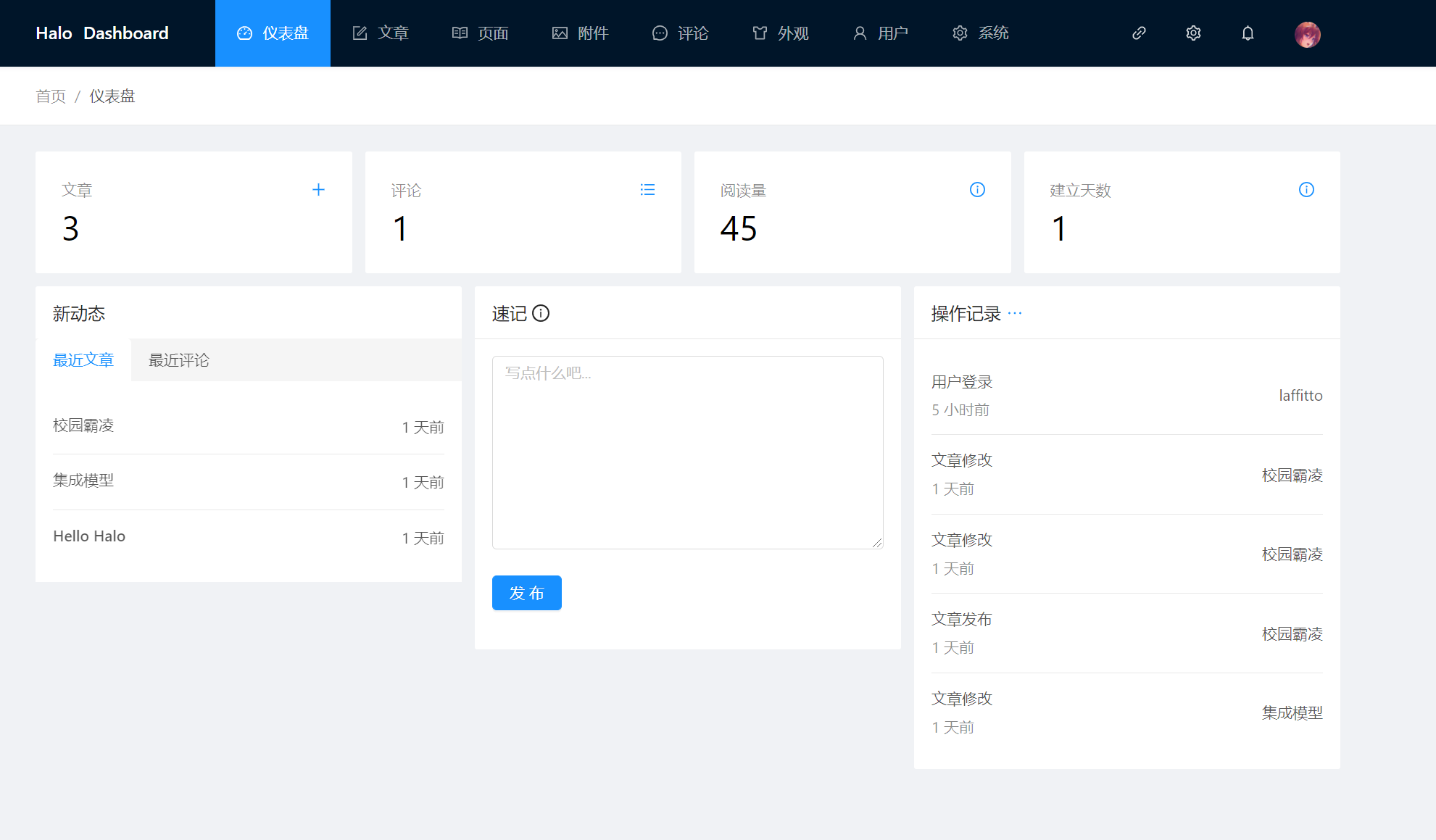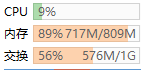]]>
<![CDATA[校园霸凌]]> tag:https://laffitto.xyz,2021-06-08:xiao-yuan-ba-ling 2021-06-08T20:13:01+08:00 2021-06-27T20:14:52+08:00 laffitto https://laffitto.xyz 一些虚幻与现实的回想

]]>
<![CDATA[集成模型]]> tag:https://laffitto.xyz,2021-05-22:集成模型 2021-05-22T20:11:57+08:00 2022-03-25T15:15:50+08:00 laffitto https://laffitto.xyz 集成模型简单的总结，后续有时间再继续完善

• Bagging：这类方法的代表是随机森林
• Boosting：这类方法的代表是GBDT和XGBoost

## Bagging

Bagging主要是通过平均带来方差的减少（variance reduction）。假设有N个模型，每个模型再预测时的方差为 $\sigma^2$ 。则通过N个模型一起预测时的方差是（平均）：$\frac{\sigma^2} N$

Random Forests = Bagging w. trees + random feature subsets

## Boosting

Boosting算法本质是通过学习一个个弱分类器，在之前分类器的基础上不断迭代，最终集成提升为强分类器。boosting方法大致可以分为两种：

### CART回归树

GBDT使用的决策树基础是CART回归树，由于其每次迭代要拟合的是梯度值，属于连续值，所以不可以使用CART分类树。为了很好的评判拟合程序，选择使用平方误差（MSE）。

1. 选择最优切分变量$j$与切分点$s$，求解

$\min _{j, s}\left[\min _{c_{1}} \sum_{x_{i} \in R_{1}(j, s)}\left(y_{i}-c_{1}\right)^{2}+\min _{c_{2}} \sum_{x_{i} \in R_{2}(j, s)}\left(y_{i}-c_{2}\right)^{2}\right]$

1. 用选定的对$(j,s)$划分区域并决定相应的输出值：

$R_{1}(j, s)=x\left|x^{(j)} \leq s, R_{2}(j, s)=x\right| x^{(j)}>s$

$\hat{c_{m}}=\frac{1}{N} \sum_{x_{1} \in R_{m}(j, s)} y_{i}, x \in R_{m}, m=1,2$

1. 继续对两个子区域调用步骤1和2，直至满足停止条件（特定迭代次数或值满足某些条件）。
2. 将输入空间划分为$M$个区域 $R_1,R_2,...R_M$ ，生成决策树：

$f(x)=\sum_{m=1}^{M} \hat{c}_{m} I\left(x \in R_{m}\right)$

### 提升树

1. 初始化$f_0(x)=0$

2. For $m=1,2,……M$

• 针对每个样本$(x_i,y_i)$，计算残差

$r _ { m , i } = y _ { i } - f _ { m - 1 } ( x _ { i } ) , i = 1 , 2 , \cdots , N$

• 利用 $\left\{\left(x_{i}, r_{m, i}\right)\right\}_{i=1,2, \ldots, N}$ 训练出一个决策树（回归树），得到 $T ( x ; \Theta _ { m } )$

• 更新$f_{m}(x)=f_{m-1}(x)+T\left(x ; \Theta_{m}\right)$

1. 完成以上迭代，即可得到提升树

$f_{M}(x)=\sum_{m=1}^{M} T\left(x ; \Theta_{m}\right)$

### GBDT

GBDT是基于 Boosting的思想，串行地构造多棵决策树来进行数据的预测，对损失函数做梯度下降，每轮迭代都去拟合损失函数在当前模型下的负梯度，把待求的決策树模型当作参数，从而使得参数朝着最小化损失函数的方向更新。

GBDT算法

1. 初始化 $f_{0}(x)=\arg \min _{c} \sum_{i=1}^{N} L\left(y_{i}, c\right)$

2. For $m=1,2,……M$

• 针对每个样本$(x_i,y_i)$，计算残差

$r_{m, i}=-\left[\frac{\partial L\left(y_{i}, f\left(x_{i}\right)\right)}{\partial f\left(x_{i}\right)}\right]_{ \left.f(x)=f_{m-1}(x)\right)}, i=1,2, \ldots, N$

• 利用$\left\{\left(x_{i}, r_{m, i}\right)\right\}_{i=1,2, \ldots, N}$ ,训练出第$m$棵回归树$T_m$，其叶节点划分的区域为$R_{m, j}, j=1,2, \ldots, J$

• 对于回归树的每一个叶节点，计算器输出值

$c_{m, j}=\arg \min _{c} \sum_{x_{i} \in R_{m, j}} L\left(y_{i}, f_{m-1}\left(x_{i}\right)+c\right), j=1,2, \ldots, J$

• 更新$f_{m}(x)=f_{m-1}(x)+\sum_{j=1}^{J} c_{m, j} I\left(x \in R_{m, j}\right)$
1. 得到最终的梯度提升回归树

$\hat{f}(x)=f_{M}(x)=\sum_{m=1}^{M} \sum_{j=1}^{J} c_{m, j} I\left(x \in R_{m, j}\right)$

### XGBoost

XGBoost是对GBDT的进一步改进。传统GBDT在优化时只用到一阶导数信息， Xgboost则对损失函数进行了二阶泰勒展开，同时用到了一阶和二阶导数。
XGBOOST在损失函数里加入了正则项，用于控制模型的复杂度。正则项里包含了树的叶子节点个数、每个叶子节点上输出的 score的L2模的平方和。从 Bias-variance tradeoff角度来讲，正则项降低了模型的 variance,使学习出来的模型更加简单，防止过拟合，这也是 Xgboost优于传统GBDT的一个特性。

1. 如何构造目标函数

$\hat{y}_{i}=\phi\left(X_{i}\right)=\sum_{k=1}^{K} f_{k}\left(X_{i}\right), f_{k} \in \mathcal{F}$

$\mathcal{L}(\phi)=\sum_{i} l\left(\hat{y}_{i}, y_{i}\right)+\sum_{k} \Omega\left(f_{k}\right)$

$\hat{k}_{i}^{(t)}=\hat{k}_{i}(t-1)+f_{k}\left(x_{i}\right)$

\begin{aligned} \mathcal{L}(\phi) &=\sum_{i}^{n} l\left(y_{i}, \hat{y}_{i}^{(k)}\right)+\sum_{k=1}^{K} \Omega\left(f_{k}\right) \\ &=\sum_{i}^{n} l\left(y_{i}, \hat{y}_{i}^{(k-1)}+f_{k}\left(X_{i}\right)\right)+\sum_{k=1}^{K-1} \Omega\left(f_{K}\right)+\Omega\left(f_{K}\right)\end{aligned}

\begin{aligned} \mathcal{L}^{(K)} &=\sum_{i}^{n} l\left(y_{i}, \hat{y}_{i}^{(k-1)}+f_{k}\left(X_{i}\right)\right)+\Omega\left(f_{K}\right)\end{aligned}

1. 目标函数的近似

$f ( x + \Delta x ) \approx f ( x ) + f ^ { \prime } ( x ) \Delta x + \frac { 1 } { 2 } f ^ { \prime \prime } ( x ) \Delta x ^ { 2 }$

$f_k(X_i)$看作$\Delta x$（为何可以这样？因为每棵树迭代相加提升的数值很小，可以这样去理解）。则第K棵树目标函数转化为：

$\mathcal{L}^{(K)} \simeq \sum_{i=1}^{n}\left[l\left(y_{i}, \hat{y}_{i}^{(K-1)}\right)+g_{i} f_{K}\left(X_{i}\right)+\frac{1}{2} h_{i} f_{K}^{2}\left(X_{i}\right)\right]+\Omega\left(f_{K}\right)$

1. 将树的结构引入目标函数

$\Omega\left(f_{K}\right)=\gamma T+\frac{1}{2} \lambda \sum_{j=1}^{T} w_{j}^{2}$

\begin{aligned} \mathcal{L}^{(K)} & \simeq \sum_{i=1}^{n}\left[g_{i} f_{K}\left(X_{i}\right)+\frac{1}{2} h_{i} f_{K}^{2}\left(X_{i}\right)\right]+\Omega\left(f_{K}\right) \\ &=\sum_{i=1}^{n}\left[g_{i} w_{q\left(x_{i}\right)}+\frac{1}{2} h_{i} w_{q\left(x_{i}\right)}^{2}\right]+\gamma T+\lambda \frac{1}{2} \sum_{j=1}^{T} w_{j}^{2} \\ &=\sum_{j=1}^{T}\left[\left(\sum_{i \in I_{j}} g_{i}\right) w_{j}+\frac{1}{2}\left(\sum_{i \in I_{j}} h_{i}+\lambda\right) w_{j}^{2}\right]+\gamma T \end{aligned}

Tips:第二至三步：将所有节点1到n的循环改写成单独叶节点$I_J$1到T的循环（优点：去除下标，包含二次项）

$G_{j}=\sum_{i \in I_{j}} g_{i}, H_{j}=\sum_{i \in I_{j} }g_{i}$，代入上式：

$\mathcal{L}^{(K)}=\sum_{j=1}^{T}\left[G_{j} w_{j}+\frac{1}{2}\left(H_{j}+\lambda\right) w_{j}^{2}\right]+\gamma T$

$w _ { j } ^ { * } = - \frac { G _ { j } } { H _ { j } + \lambda }$

$L ^ { ( K ) } = - \frac { 1 } { 2 } \sum _ { j = 1 } ^ { T } \frac { G _ { j } ^ { 2 } } { H _ { j } + \lambda } + \gamma T$

1. 优化方法（使用贪心算法）

$G a i n=\frac{1}{2}\left[\frac{G_{L}^{2}}{H_{L}+\lambda}+\frac{G_{R}^{2}}{H_{R}+\lambda}-\frac{\left(G_{L}+G_{R}\right)^{2}}{H_{L}+H_{R}+\lambda}\right]-\gamma$

$\mathcal{L}_{\text {split }}=\frac{1}{2}\left[\frac{\left(\sum_{i \in I_{L}} g_{i}\right)^{2}}{\sum_{i \in I_{L}} h_{i}+\lambda}+\frac{\left(\sum_{i \in I_{R}} g_{i}\right)^{2}}{\sum_{i \in I_{R}} h_{i}+\lambda}-\frac{\left(\sum_{i \in I} g_{i}\right)^{2}}{\sum_{i \in I} h_{i}+\lambda}\right]-\gamma$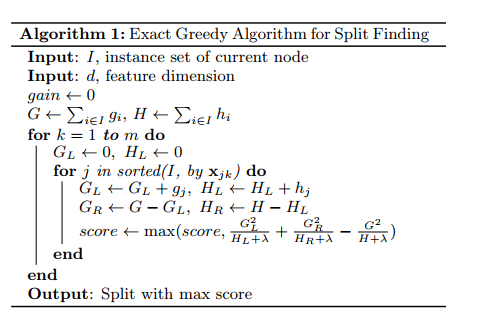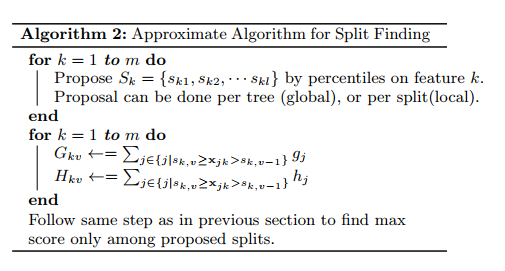XGBoost同样有很多优化，例如Shrinkage，列抽样，稀疏值处理等等，也不细展开了，具体详见论文。

GBDT算法原理以及实例理解

XGBoost论文解读